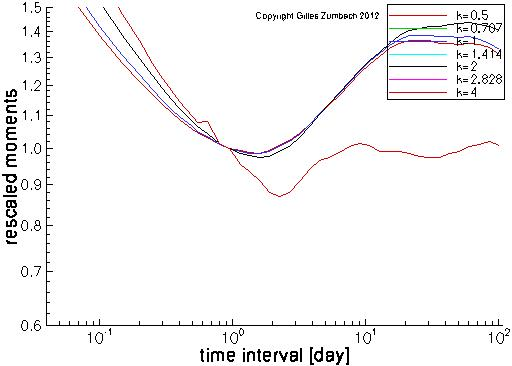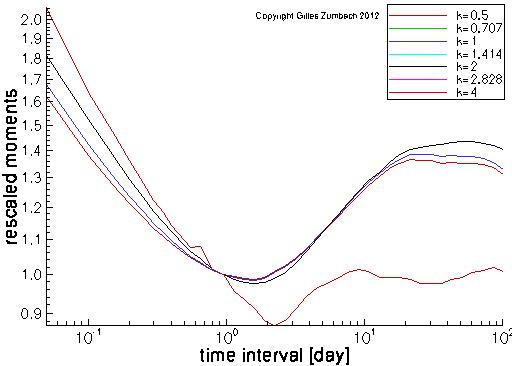Base information Indexes

# Scaling for the moments for "GARCH(1,1)"

## Normalized moments of the return

The horizontal axis gives the time horizons of the return, the vertical axis gives the normalized moments.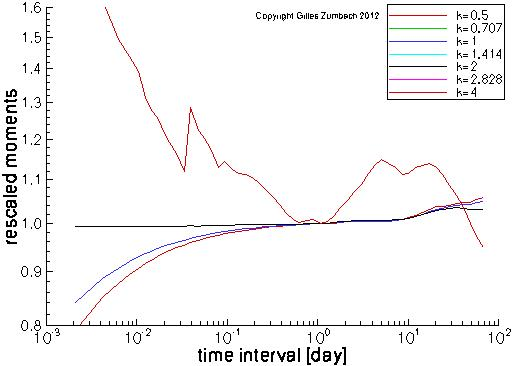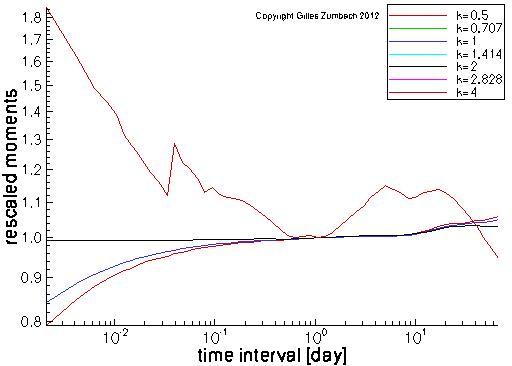## Normalized moments of the volatility

The horizontal axis gives the time horizons of the volatility, the vertical axis gives the normalized moments.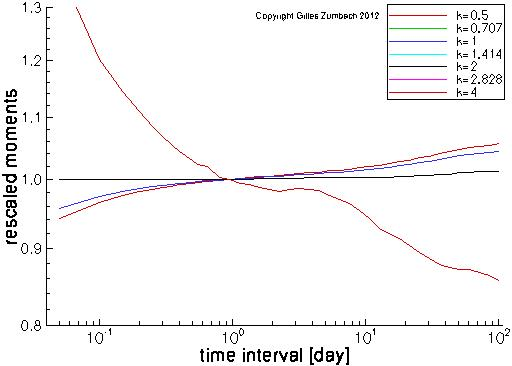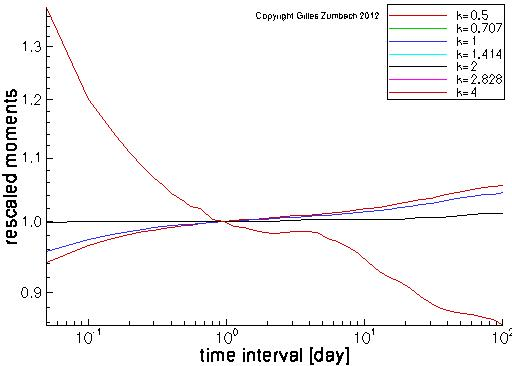## Normalized moments of the volatility increment

The horizontal axis gives the time horizons of the volatility increment, the vertical axis gives the normalized moments.